SSC CGL Previous Year Questions: Ratio, Proportion, Mixture and Partnership - 2

# SSC CGL Previous Year Questions: Ratio, Proportion, Mixture and Partnership - 2 - SSC CGL

Test Description

## 30 Questions MCQ Test SSC CGL (Tier - 1) - Previous Year Papers (Topic Wise) - SSC CGL Previous Year Questions: Ratio, Proportion, Mixture and Partnership - 2

SSC CGL Previous Year Questions: Ratio, Proportion, Mixture and Partnership - 2 for SSC CGL 2023 is part of SSC CGL (Tier - 1) - Previous Year Papers (Topic Wise) preparation. The SSC CGL Previous Year Questions: Ratio, Proportion, Mixture and Partnership - 2 questions and answers have been prepared according to the SSC CGL exam syllabus.The SSC CGL Previous Year Questions: Ratio, Proportion, Mixture and Partnership - 2 MCQs are made for SSC CGL 2023 Exam. Find important definitions, questions, notes, meanings, examples, exercises, MCQs and online tests for SSC CGL Previous Year Questions: Ratio, Proportion, Mixture and Partnership - 2 below.
Solutions of SSC CGL Previous Year Questions: Ratio, Proportion, Mixture and Partnership - 2 questions in English are available as part of our SSC CGL (Tier - 1) - Previous Year Papers (Topic Wise) for SSC CGL & SSC CGL Previous Year Questions: Ratio, Proportion, Mixture and Partnership - 2 solutions in Hindi for SSC CGL (Tier - 1) - Previous Year Papers (Topic Wise) course. Download more important topics, notes, lectures and mock test series for SSC CGL Exam by signing up for free. Attempt SSC CGL Previous Year Questions: Ratio, Proportion, Mixture and Partnership - 2 | 30 questions in 30 minutes | Mock test for SSC CGL preparation | Free important questions MCQ to study SSC CGL (Tier - 1) - Previous Year Papers (Topic Wise) for SSC CGL Exam | Download free PDF with solutions
 1 Crore+ students have signed up on EduRev. Have you?
SSC CGL Previous Year Questions: Ratio, Proportion, Mixture and Partnership - 2 - Question 1

### What must be added to each term of the ratio 2 : 5 so that it may equal to 5 : 6?    (SSC CGL 1st Sit. 2015)

Detailed Solution for SSC CGL Previous Year Questions: Ratio, Proportion, Mixture and Partnership - 2 - Question 1

According to question,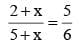⇒ 12 + 6x = 25 + 5x
⇒ x = 25  – 12 = 13.

SSC CGL Previous Year Questions: Ratio, Proportion, Mixture and Partnership - 2 - Question 2

### 729 ml of a mixture contains milk and water in the ratio 7 : 2. How much more water is to be added to get a new mixture containing milk and water in the ratio 7 : 3?    (SSC CGL 1st Sit. 2015)

Detailed Solution for SSC CGL Previous Year Questions: Ratio, Proportion, Mixture and Partnership - 2 - Question 2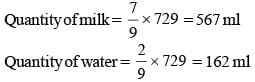Let ‘x’ be the quantity that should be added to make the ratio 7 : 3
According to the question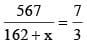⇒ 1701  = 1134 + 7x
⇒ 7x  = 1701 – 1134
⇒ x = 81 ml

SSC CGL Previous Year Questions: Ratio, Proportion, Mixture and Partnership - 2 - Question 3

### Eighteen years ago, the ratio of A's age to B's age was 8 : 13. Their present age ratio's are 5 : 7. What is the present age of A?    (SSC CGL 1st Sit.  2015)

Detailed Solution for SSC CGL Previous Year Questions: Ratio, Proportion, Mixture and Partnership - 2 - Question 3

Let the A’s age and B’s age was 8x and 13x
According to question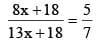⇒ 56x + 18 x 7  = 65x + 18 x 5
⇒ 65x – 56x  = 18 x 7 – 18 x 5
⇒ 9x  = 18 x 2
⇒ x  = 4
Hence, the present age of A = 8 x 4 + 18 = 50 yrs.

SSC CGL Previous Year Questions: Ratio, Proportion, Mixture and Partnership - 2 - Question 4

A vessel contains 60 litres of milk. 12 litres of milk taken out from it and replaced by water. Then again from mixture, 12 litres are again taken out and replaced by water. The ratio of milk and  water in the resultant mixture is:    (SSC CHSL 1st Sit. 2015)

Detailed Solution for SSC CGL Previous Year Questions: Ratio, Proportion, Mixture and Partnership - 2 - Question 4

12l of milk taken out of 60l milk. So, 20% water is added to milk
Milk = 48l ; Water = 12l
Now, again 20% water is added to this mixture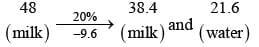So, ratio of milk and water = 38.4 : 21.6 = 16 : 9
Alternate Method:
Final Volume of milk = Initial volume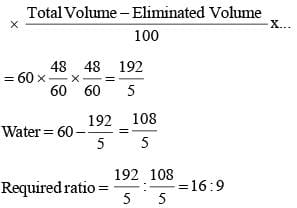SSC CGL Previous Year Questions: Ratio, Proportion, Mixture and Partnership - 2 - Question 5

The current ages of Sonali and Monali are in the ratio 5 : 3. Five years from now, their ages will be in the ratio 10 : 7. Then, Monali's current age is:    (SSC CHSL 1st Sit. 2015)

Detailed Solution for SSC CGL Previous Year Questions: Ratio, Proportion, Mixture and Partnership - 2 - Question 5

Ratio of present ages of Sonali and Monali = 5 : 3
After 5 years, ratio of ages of both girls = 10 : 7
Let actual present ages are 5x and 3x years.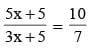⇒ 35x + 35 = 30x + 50
⇒ 5x = 15
⇒ x = 3
So, Monali age = 3 × 3 = 9 years

SSC CGL Previous Year Questions: Ratio, Proportion, Mixture and Partnership - 2 - Question 6

Incomes of x and y are in the ratio 4 : 3. Their expenditures are in the ratio 12 : 7. Both save ₹ 3200 at the end of the month, then the income of x is:    (SSC Sub. Ins. 2015)

Detailed Solution for SSC CGL Previous Year Questions: Ratio, Proportion, Mixture and Partnership - 2 - Question 6

Ratio of income of x and y = 4 : 3
Ratio of expenditure of x and y = 12 : 7
Saving of x = 4a – 12b = 3200 ...(i)
Saving of y = 3a – 7b= 3200 ..(ii)
Solving (i) and (ii)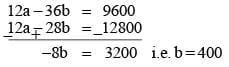Now 3a = 3200 + 7b = 3200 + 7 (400) = 6000 a = ₹ 2000
So income of x = ₹ 2000 × 4 = 8000

SSC CGL Previous Year Questions: Ratio, Proportion, Mixture and Partnership - 2 - Question 7

Gold is 19 times as heavy as water and copper is 9 times as heavy as water. In what ratio should these be mixed to get an alloy 15 times as heavy as water?    (SSC CGL 1st Sit.  2014)

Detailed Solution for SSC CGL Previous Year Questions: Ratio, Proportion, Mixture and Partnership - 2 - Question 7

By rule of alligation, we have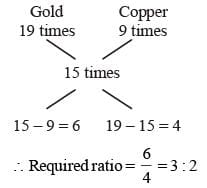SSC CGL Previous Year Questions: Ratio, Proportion, Mixture and Partnership - 2 - Question 8

If x : y = 5 : 2, then (8x + 9y) : (8x + 2y) is    (SSC CHSL 2nd Sit.  2014)

Detailed Solution for SSC CGL Previous Year Questions: Ratio, Proportion, Mixture and Partnership - 2 - Question 8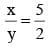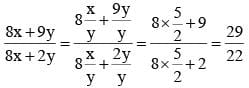SSC CGL Previous Year Questions: Ratio, Proportion, Mixture and Partnership - 2 - Question 9

Two numbers are in the ratio 3 : 5. If 9 is subtracted from each, the new numbers are in the ratio 12 : 23. The small number is    (SSC CHSL 2nd Sit.  2014)

Detailed Solution for SSC CGL Previous Year Questions: Ratio, Proportion, Mixture and Partnership - 2 - Question 9

Let two numbers be 3x and 5x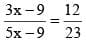⇒ 23(3x – 9) = 12(5x – 9)
⇒ 69x – 207 = 60x – 108
⇒ 9x = 99
⇒ x = 11
Hence, the small number will be 3 ×11 = 33

SSC CGL Previous Year Questions: Ratio, Proportion, Mixture and Partnership - 2 - Question 10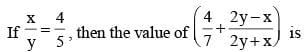(SSC CHSL 1st Sit.  2014)

Detailed Solution for SSC CGL Previous Year Questions: Ratio, Proportion, Mixture and Partnership - 2 - Question 10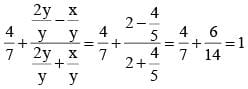SSC CGL Previous Year Questions: Ratio, Proportion, Mixture and Partnership - 2 - Question 11

₹ 730 were divided among A, B, C in such a way that if A gets ₹ 3, then B gets ₹ 4 and if B gets ₹ 3.50 then C gets ₹ 3.
The share of B exceeds that of C by    (SSC Sub. Ins. 2014)

Detailed Solution for SSC CGL Previous Year Questions: Ratio, Proportion, Mixture and Partnership - 2 - Question 11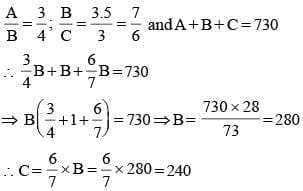B exceeds that of C by (280 – 240) = ₹ 40

SSC CGL Previous Year Questions: Ratio, Proportion, Mixture and Partnership - 2 - Question 12

If x : y :: 2 : 3 and 2 : x :: 4 : 8, then the value of y is    (SSC Sub. Ins. 2014)

Detailed Solution for SSC CGL Previous Year Questions: Ratio, Proportion, Mixture and Partnership - 2 - Question 12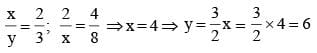SSC CGL Previous Year Questions: Ratio, Proportion, Mixture and Partnership - 2 - Question 13

A box contain 280 coins of one rupee, 50 paise and 25 paise. The values of each kind of coin are in the ratio of 8 : 4 : 3. The number of one rupee coins will be    (SSC Multitasking 2014)

Detailed Solution for SSC CGL Previous Year Questions: Ratio, Proportion, Mixture and Partnership - 2 - Question 13

Ratio of number of coins
= 8 : 4 × 2 : 3 × 4 = 8 : 8 : 12  = 2 : 2 : 3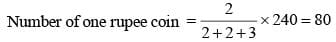SSC CGL Previous Year Questions: Ratio, Proportion, Mixture and Partnership - 2 - Question 14

The ratio of the ages of A, B and C is 5 : 8 : 9. If the sum of the ages of A and C is 56 years, the age of B will be    (SSC Multitasking 2014)

Detailed Solution for SSC CGL Previous Year Questions: Ratio, Proportion, Mixture and Partnership - 2 - Question 14

Let ages of A, B and C are 5x, 8x and 9x respectively.
∴ 5x + 9x = 56 ⇒ x = 4
∴ Age of B = 8 × 4 = 32 years

SSC CGL Previous Year Questions: Ratio, Proportion, Mixture and Partnership - 2 - Question 15

If 2x = 3y = 4z, find x : y : z.    (SSC Multitasking 2014)

Detailed Solution for SSC CGL Previous Year Questions: Ratio, Proportion, Mixture and Partnership - 2 - Question 15

2x = 3y = 4z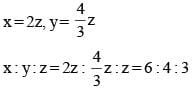SSC CGL Previous Year Questions: Ratio, Proportion, Mixture and Partnership - 2 - Question 16

The ratio of 252.5 : 53 is same as    (SSC CGL 1st Sit.  2013)

Detailed Solution for SSC CGL Previous Year Questions: Ratio, Proportion, Mixture and Partnership - 2 - Question 16

(52)2.5 = 55 : 53 = 52 : 1 = 25 : 1

SSC CGL Previous Year Questions: Ratio, Proportion, Mixture and Partnership - 2 - Question 17

Divide 81 into three parts so that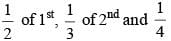of 3rd are equal.    (SSC CGL 1st Sit.  2013)

Detailed Solution for SSC CGL Previous Year Questions: Ratio, Proportion, Mixture and Partnership - 2 - Question 17

Let 1st, 2nd and 3rd part represented by x, y, z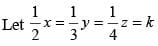∴ x = 2k, y = 3k, z = 4k
According to question
x + y + z = 81
⇒ 2k + 3k + 4k = 81
⇒ 9k = 81
⇒ k = 9
Hence, parts are 18, 27, 36.

SSC CGL Previous Year Questions: Ratio, Proportion, Mixture and Partnership - 2 - Question 18

The prize money of ₹ 1,800 is divided among 3 students A, B and C in such a way that 4 times the share of A is equal to 6 times the share of B, which is equal to 3 times the share of C. Then A’s share is    (SSC CGL 1st Sit.  2013)

Detailed Solution for SSC CGL Previous Year Questions: Ratio, Proportion, Mixture and Partnership - 2 - Question 18

4A = 6B ⇒ 2A = 3B ⇒ A : B = 3 : 2
6B = 3C ⇒ 2 B = C ⇒ B : C = 1 : 2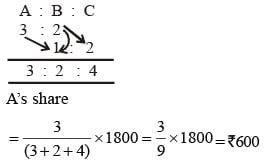Shortcut Method:
4A = 6B = 3C
A : B : C = 18 : 12 : 24 = 3 : 2 : 4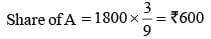SSC CGL Previous Year Questions: Ratio, Proportion, Mixture and Partnership - 2 - Question 19

A sum of ₹ 300 is divided among P, Q and R in such a way that Q gets ₹ 30 more than P and R gets ₹ 60 more than Q.
The ratio of their share is    (SSC CGL 1st Sit.  2013)

Detailed Solution for SSC CGL Previous Year Questions: Ratio, Proportion, Mixture and Partnership - 2 - Question 19

Q = P + 30 ⇒ Q – P = 30 and
R – Q = 60 = 2 × 30
∴ Required ratio = 2 : 3 : 5
Look : 3 – 2 = 1, 5 – 3 = 2

SSC CGL Previous Year Questions: Ratio, Proportion, Mixture and Partnership - 2 - Question 20

The prices of a school bag and a shoe are in the ratio 7 : 5. The price of a school bag is ₹ 200 more than the price of a shoe.
Then the price of a shoe is    (SSC CGL 1st Sit.  2013)

Detailed Solution for SSC CGL Previous Year Questions: Ratio, Proportion, Mixture and Partnership - 2 - Question 20

7x – 5x = 200
⇒ 2x = 200 ⇒ x = 100
∴ Price of a pair of shoes = 5x = ₹ 500

SSC CGL Previous Year Questions: Ratio, Proportion, Mixture and Partnership - 2 - Question 21

15 litres of a mixture contains alcohol and water in the ratio 1 : 4. If 3 litres of water is mixed in it, the percentage of alcohol in the new mixture will be    (SSC CGL 2nd Sit.  2013)

Detailed Solution for SSC CGL Previous Year Questions: Ratio, Proportion, Mixture and Partnership - 2 - Question 21

Alcohol = 3 litres
Water = 12 litres
∴ Required percentage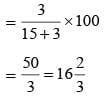SSC CGL Previous Year Questions: Ratio, Proportion, Mixture and Partnership - 2 - Question 22

A and B have together three times what B and C have, while A, B, C together have thirty rupees more than that of A. If B has 5 times that of C, then A has     (SSC CGL 2nd Sit.  2013)

Detailed Solution for SSC CGL Previous Year Questions: Ratio, Proportion, Mixture and Partnership - 2 - Question 22

A + B = 3 (B + C)
A + B + C = A + 30
B = 5C
∴ A + B = 3 (B  + C)
⇒ A + 5C = 18C ⇒ A = 13C
∴ A + B + C = A + 30
⇒ A + 5C + C = A + 30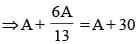⇒ 6A = 30 × 13
⇒ A = ₹ 65
Alternate Method:
Let C = C B = 5C
A + B = 3 (B + C)
A + 5C = 3 × 6 C ∴ A = 13 C
A + B + C  = A + 30
SC + C = 30 ∴ C = 15
A = 13 ×5  = 65

SSC CGL Previous Year Questions: Ratio, Proportion, Mixture and Partnership - 2 - Question 23

The ratio of monthly incomes of A, B is 6 : 5 and their monthly expenditures are in the ratio 4 : 3. If each of them saves ₹ 400 per month, find the sum of their monthly incomes.    (SSC CGL 2nd Sit.  2013)

Detailed Solution for SSC CGL Previous Year Questions: Ratio, Proportion, Mixture and Partnership - 2 - Question 23

Incomes of A and B
= ₹ 6x and ₹ 5x
Expenses of A and B
= ₹ 4y and ₹ 3y
∴ 6x – 4y = 400 ...(i)
5x – 3y = 400 ...(ii)
By equation (i) × 3 – (ii) × 4
⇒ 18x – 12y – 20x + 12y
= 1200 – 1600
⇒ 2x = 400 ⇒ x = 200
∴ Total income
= 6x + 5x = 11x = ₹ 2200

SSC CGL Previous Year Questions: Ratio, Proportion, Mixture and Partnership - 2 - Question 24

₹ 700 is divided among A, B, C in such a way that the ratio of the amount of A and B is 2 : 3 and that of B and C is 4 : 5.
Find the amounts in ₹ each received, in the order A, B, C.    (SSC CGL 2nd Sit.  2013)

Detailed Solution for SSC CGL Previous Year Questions: Ratio, Proportion, Mixture and Partnership - 2 - Question 24

A : B = 2 : 3 = 8 : 12
B : C = 4 : 5 = 12 : 15
∴ A : B : C = 8 : 12 : 15
Sum of ratio = 35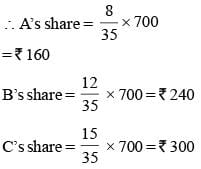SSC CGL Previous Year Questions: Ratio, Proportion, Mixture and Partnership - 2 - Question 25

Three numbers are in the ratio 1 : 2 : 3. By adding 5 to each of them, the new numbers are in the ratio 2 : 3 : 4. The numbers are:    (SSC CGL 1st Sit.  2013)

Detailed Solution for SSC CGL Previous Year Questions: Ratio, Proportion, Mixture and Partnership - 2 - Question 25

Numbers = x, 2x and 3x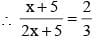⇒  4x +10 = 3x +15
⇒  x = 5
⇒ Number = 5, 10 and 15

SSC CGL Previous Year Questions: Ratio, Proportion, Mixture and Partnership - 2 - Question 26

A invests ₹ 64,000 in a business. After few months, B joined him with ₹ 48,000. At the end of year, the total profit was divided between them in the ratio 2 : 1. After how many months did B join?    (SSC CHSL  2nd Sit.  2013)

Detailed Solution for SSC CGL Previous Year Questions: Ratio, Proportion, Mixture and Partnership - 2 - Question 26

Suppose, B Joined after x month
Then B's money was invested for (12 – x) months
∴ According to question,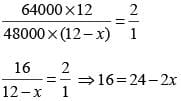⇒ 2x = 24 – 16 ⇒ x = 4
Hence, B joined after 4 months

SSC CGL Previous Year Questions: Ratio, Proportion, Mixture and Partnership - 2 - Question 27

The ratio of age of two boys is 5 : 6. After two years, the ratio will be 7 : 8. The ratio of their ages after 12 years will be    (SSC CHSL 1st Sit.  2013)

Detailed Solution for SSC CGL Previous Year Questions: Ratio, Proportion, Mixture and Partnership - 2 - Question 27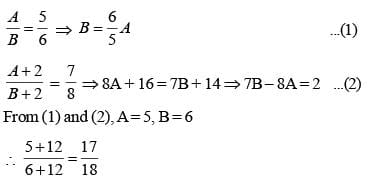SSC CGL Previous Year Questions: Ratio, Proportion, Mixture and Partnership - 2 - Question 28

The proportion of acid and water in three samples is 2 : 1, 3 : 2 and 5 : 3. A mixture containing equal quantities of all three samples is made. The ratio of water and acid in the mixture is:    (SSC Sub. Ins. 2013)

Detailed Solution for SSC CGL Previous Year Questions: Ratio, Proportion, Mixture and Partnership - 2 - Question 28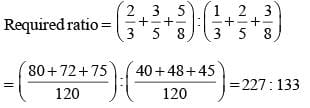SSC CGL Previous Year Questions: Ratio, Proportion, Mixture and Partnership - 2 - Question 29

A man ordered 4 pairs of black socks and some pairs of brown socks. The price of a black socks is double that of a brown pair. While preparing the bill the clerk interchanged the number of black and brown pairs by mistake which increased the bill by 50%. The ratio of the number of black and brown pairs of socks in the original order was:    (SSC Sub. Ins. 2013)

Detailed Solution for SSC CGL Previous Year Questions: Ratio, Proportion, Mixture and Partnership - 2 - Question 29

Number of brown socks = x
Price of brown socks = Rs. y per pair
Price of black socks = Rs. 2y per pair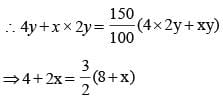⇒ 8 + 4x = 24 + 3x
⇒ x = 24 – 8 = 16
∴ Requried ratio = 4 : 16 = 1 : 4

SSC CGL Previous Year Questions: Ratio, Proportion, Mixture and Partnership - 2 - Question 30

The present ages of two persons are 36 and 50 years respectively, if after n years the ratio of their ages will be 3 : 4, then the value of n is    (SSC Multitasking 2013)

Detailed Solution for SSC CGL Previous Year Questions: Ratio, Proportion, Mixture and Partnership - 2 - Question 30

According to question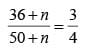⇒ 36 × 4 + 4n = 50 × 3 + 3n
⇒ 4n – 3n = 150 – 144
⇒ n = 6

## SSC CGL (Tier - 1) - Previous Year Papers (Topic Wise)

250 tests
Information about SSC CGL Previous Year Questions: Ratio, Proportion, Mixture and Partnership - 2 Page
In this test you can find the Exam questions for SSC CGL Previous Year Questions: Ratio, Proportion, Mixture and Partnership - 2 solved & explained in the simplest way possible. Besides giving Questions and answers for SSC CGL Previous Year Questions: Ratio, Proportion, Mixture and Partnership - 2, EduRev gives you an ample number of Online tests for practice

250 tests